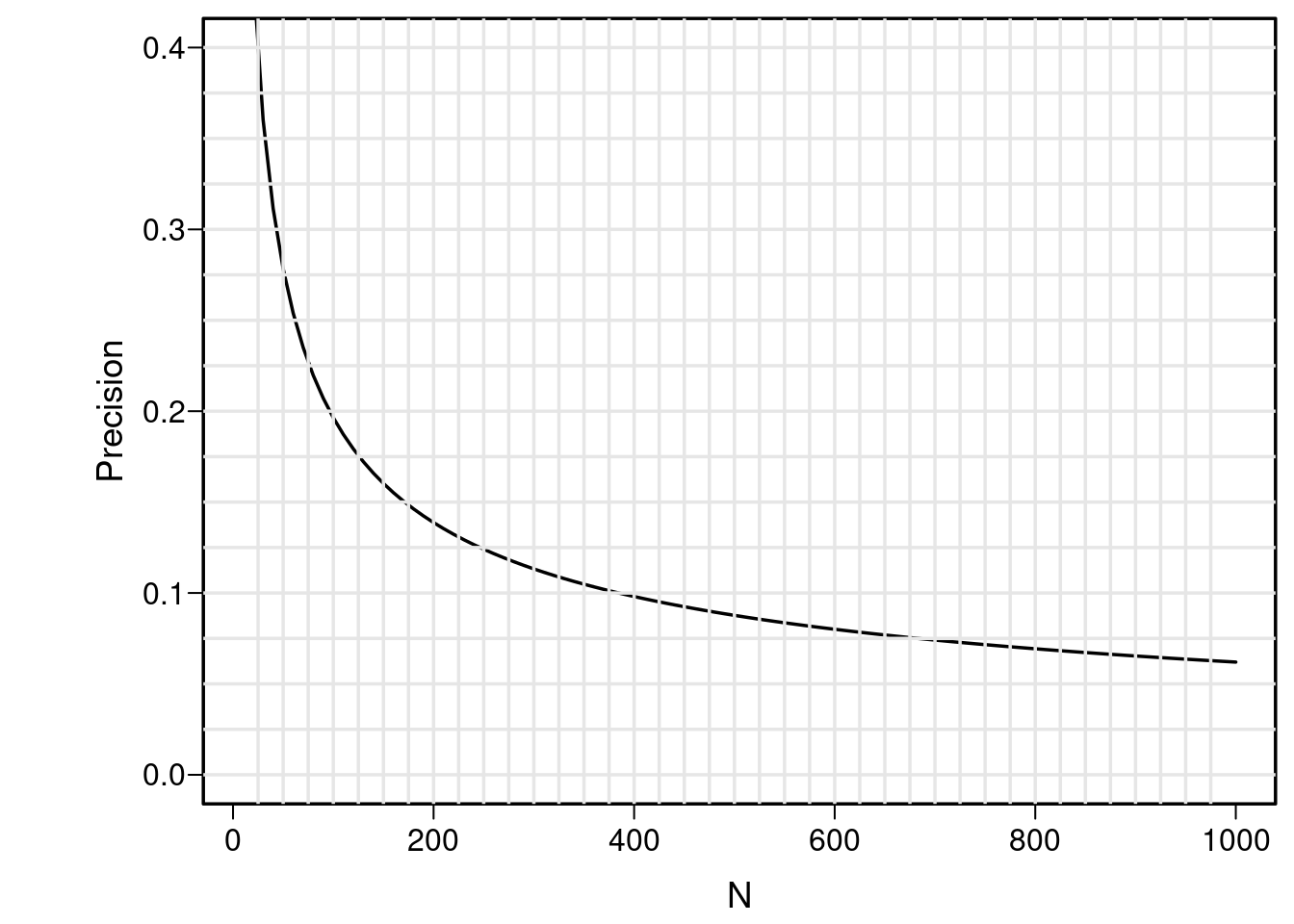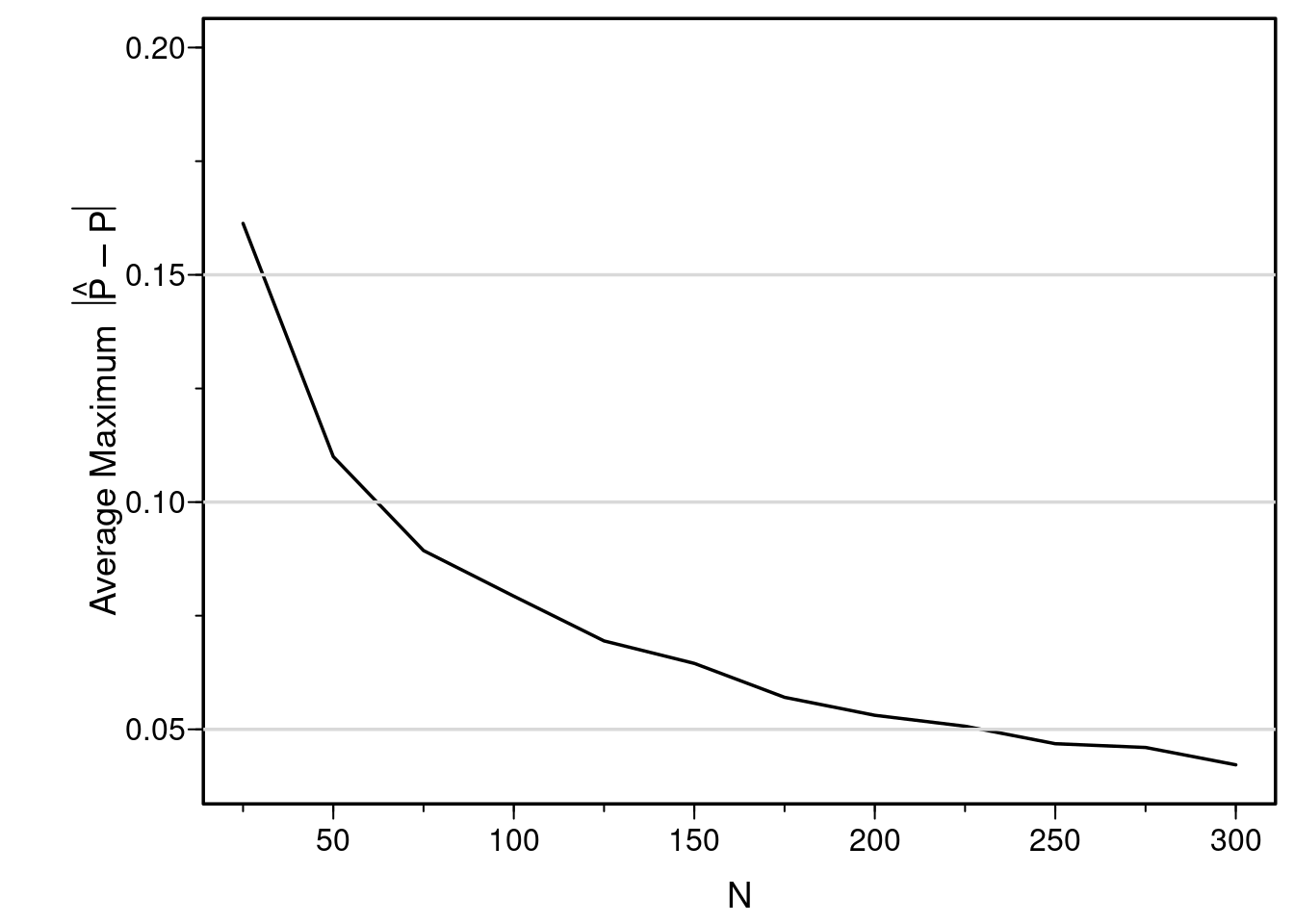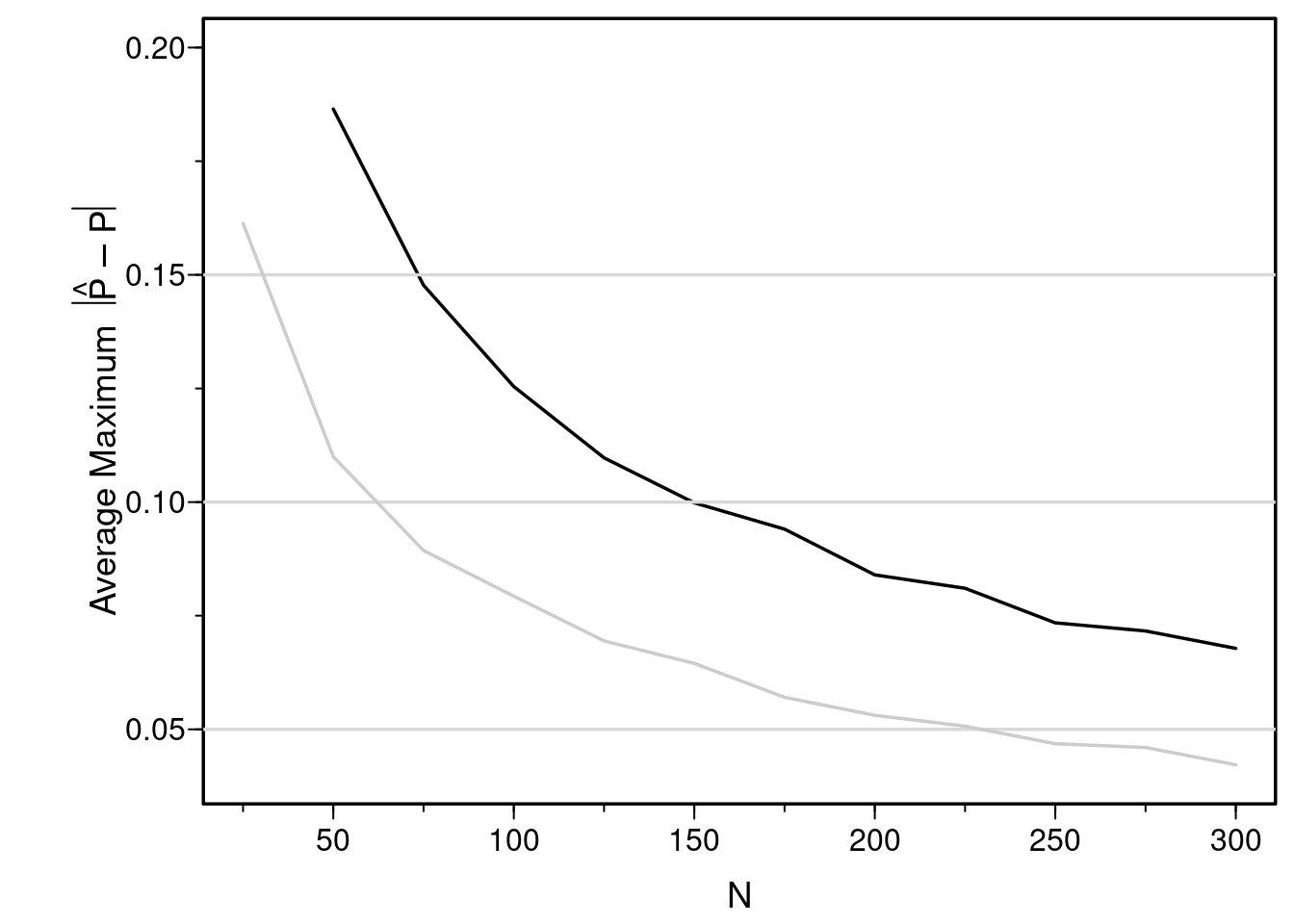# How Can Machine Learning be Reliable When the Sample is Adequate for Only One Feature?

prediction
machine-learning
sample-size
validation
precision
accuracy-score
2018
It is easy to compute the sample size N1 needed to reliably estimate how one predictor relates to an outcome. It is next to impossible for a machine learning algorithm entertaining hundreds of features to yield reliable answers when the sample size < N1.
Author
Affiliation

Vanderbilt University
School of Medicine
Department of Biostatistics

Published

January 11, 2018

The ability to estimate how one continuous variable relates to another continuous variable is basic to the ability to create good predictions. Correlation coefficients are unitless, but estimating them requires similar sample sizes to estimating parameters we directly use in prediction such as slopes (regression coefficients). When the shape of the relationship between X and Y is not known to be linear, a little more sample size is needed than if we knew that linearity held so that all we had to estimate was a slope and an intercept. This will be addressed later.

Consider this where it is shown that the sample size needed to estimate a correlation coefficient to within a margin of error as bad as ±0.2 with 0.95 confidence is about 100 subjects, and to achieve a better margin of error of ±0.1 requires about 400 subjects. Let’s reproduce that plot for the “hardest to estimate” case where the true correlation is 0.

Code
``````require(Hmisc)
knitrSet(lang='blogdown')``````
Code
``````plotCorrPrecision(rho=0, n=seq(10, 1000, length=100), ylim=c(0, .4), method='none')
abline(h=seq(0, .4, by=0.025), v=seq(25, 975, by=25), col=gray(.9))``````Margin for error (length of longer side of asymmetric 0.95 confidence interval) for r in estimating ρ, when ρ=0. Calculations are based on the Fisher z transformation of r.

I have seen many papers in the biomedical research literature in which investigators “turned loose” a machine learning or deep learning algorithm with hundreds of candidate features and a sample size that by the above logic is inadequate had there only been one candidate feature. How can ML possibly learn how hundreds of predictors combine to predict an outcome when our knowledge of statistics would say this is impossible? The short answer is that it can’t. Researchers claiming to have developed a useful predictive instrument with ML in the limited sample size case seldom do a rigorous internal validation that demonstrates the relationship between predicted and observed values (i.e., the calibration curve) to be a straight 45° line through the origin. I have worked with a colleague who had previously worked with a ML group who found a predictive signal (high R2) with over 1000 candidate features and N=50 subjects. In trying to check their results on new subjects we appear to be finding an R2 about 1/4 as large as originally claimed.

van der Ploeg, Austin, and Steyerberg (2014) in their article Modern modelling techniques are data hungry estimated that to have a very high chance of rigorously validating, many machine learning algorithms require 200 events per candidate feature (they found that logistic regression requires 20 events per candidate features). So it seems that “big data” methods sometimes create the need for “big data” when traditional statistical methods may not require such huge sample sizes (at least when the dimensionality is not extremely high). [Note: in higher dimensonal situations it is possible to specify a traditional statistical model for the pre-specified “important” predictors and to add in principal components and other summaries of the remaining features.] For more about “data hunger” in machine learning see this. Machine learning algorithms do seem to have unique advantages in high signal:noise ratio situations such as image and sound pattern recognition problems. Medical diagnosis and outcome prediction problems involve a low signal:noise ratio, i.e., the R2 are typically low and the outcome variable Y is typically measured with error.

I’ve shown the sample size needed to estimate a correlation coefficient with a certain precision. What about the sample size needed to estimate the whole relationship between a single continuous predictor and the probability of a binary outcome? Similar to what is presented here, let’s simulate the average maximum (over a range of X) absolute prediction error (on the probability scale). The following R program does this, for various sample sizes. 1000 simulated datasets are analyzed for each sample size considered.

Code
``````# X  = universe of X values if X considered fixed, in random order
# xp = grid of x values at which to obtain and judge predictions
require(rms)
sim <- function(assume = c('linear', 'smooth'),
X,
ns=seq(25, 300, by=25), nsim=1000,
xp=seq(-1.5, 1.5, length=200), sigma=1.5) {
assume <- match.arg(assume)
maxerr <- numeric(length(ns))
pactual <- plogis(xp)
xfixed  <- ! missing(X)

j <- 0
worst <- nsim
for(n in ns) {
j <- j + 1
maxe <- 0
if(xfixed) x <- X[1 : n]
nsuccess <- 0
for(k in 1 : nsim) {
if(! xfixed) x <- rnorm(n, 0, sigma)
P <- plogis(x)
y <- ifelse(runif(n) <= P, 1, 0)
f <- switch(assume,
linear = lrm(y ~ x),
smooth = lrm(y ~ rcs(x, 4)))
if(length(f\$fail) && f\$fail) next
nsuccess <- nsuccess + 1
phat <- predict(f, data.frame(x=xp), type='fitted')
maxe <- maxe + max(abs(phat - pactual))
}
maxe <- maxe / nsuccess
maxerr[j] <- maxe
worst <- min(worst, nsuccess)
}
if(worst < nsim) cat('For at least one sample size, could only run', worst, 'simulations\n')
list(x=ns, y=maxerr)
}
plotsim <- function(object, xlim=range(ns), ylim=c(0.04, 0.2)) {
ns <- object\$x;  maxerr <- object\$y
plot(ns, maxerr, type='l', xlab='N', xlim=xlim, ylim=ylim,
ylab=expression(paste('Average Maximum  ', abs(hat(P) - P))))
minor.tick()
abline(h=c(.05, .1, .15), col=gray(.85))
}
set.seed(1)
X <- rnorm(300, 0, sd=1.5)   # Allows use of same X's for both simulations
simrun <- TRUE
# If blogdown handled caching, would not need to manually cache with Load and Save
errLinear <- sim(assume='linear', X=X)
Save(errLinear)
}
plotsim(errLinear)``````Simulated expected maximum error in estimating probabilities for x ∈ [-1.5, 1.5] with a single normally distributed X with mean zero. The true relationship between X and P(Y=1 | X) is assumed to be logit(Y=1) = X. The logistic model fits that are repeated in the simulation assume the relationship is linear, but estimates the slope and intercept. In reality, we wouldn’t know that a relationship is linear, and if we allowed it to be nonlinear there would be a bit more variance to the estimated curve, resulting in larger average absolute errors than what are shown in the figure (see below).

But wait—the above simulation assumes that we already knew that the relationship was linear. In practice, most relationships are nonlinear but we don’t know the true transformation. Assume the relationship between X and logit(Y=1) is smooth, we can estimate the relationship reliably with a restricted cubic spline function. Here we use 4 knots, which gives rise to the addition of two nonlinear terms to the model for a total of 3 parameters to estimate not counting the intercept. By estimating these parameters we are estimating the smooth transformation of X and by simulating this process repeatedly we are allowing for “transformation uncertainty”.

Code
``````set.seed(1)
errSmooth <- sim(assume='smooth', X=X, ns=seq(50, 300, by=25))
Save(errSmooth)
}
plotsim(errSmooth, xlim=c(25, 300))
lines(errLinear, col=gray(.8))``````Estimated mean maximum (over X) absolute errors in estimating P(Y=1) when X is not assumed to predict the logit linearly (black line). The earlier estimates when linearity was assumed are shown with a gray scale line. Restricted cubic splines could not be fitted for n=25.

You can see that the sample size must exceed 300 just to have sufficient reliability in estimating probabilities just over the range of X of [-1.5, 1.5] when we do not know that the relationship is linear and we allow it to be nonlinear.

The morals of the story are

• Beware of claims of good predictive ability for ML algorithms when sample sizes are not huge in relationship to the number of candidate features
• For any problem, whether using machine learning or regression, compute the sample size needed to obtain highly reliable predictions with only a single pre-specified predictive feature
• If you are not sure that relationships are simple so that you allow various transformations to be attempted, uncertainty increases and so does the expected absolute predicton error
• If your sample size is not much bigger than the above minimum, beware of doing any high-dimensional analysis unless you have very clean data and a high signal:noise ratio
• Also remember that when Y is binary, the minimum sample size necessary just to estimate the intercept in a logistic regression model (equivalent to estimating a single proportion) is 96 (see this). So it is impossible with binary Y to accurately estimate P(Y=1 | X) when there are any candidate predictors if n < 96 (and n=96 only achives a margin of error of ±0.1 in estimating risk).
• When the number of candidate features is huge and the sample size is not, expect the list of “selected” features to be volatile, predictive discrimination to be overstated, and absolute predictive accuracy (calibration curve) to be very problematic
• In general, know how many observations are required to allow you to reliably learn from the number of candidate features you have

See here for an approach to estimating the needed sample size for a given sample size and number of candidate predictors.

# Discussion Archive (2018)

David Manheim: I agree with this point in general, but I think it’s a bit oversold - it’s not impossible to do this type of inference, it just requires additional cautions and methods appropriate for the task. And there are several methods that can be used to overcome these limitations - but I certainly agree that these methods are often not being used when people misuse the tools.

For examples of methods tailored to this particular class of task, see Liu et al’s work here; Deep Neural Networks for High Dimension, Low Sample Size Data.

Similarly, transfer learning for Neural Networks is a potentially valuable tool for learning based on a small dataset when similar tasks with larger datasets have been successful. See this.

Doing this more explicitly is possible using Bayesian methods with strong informed priors. Extending this, there are cases where the dataset can be expanded via clever use of multilevel modelling. This can use data not directly related to the question of interest to constrain the values of parameters which the domains have in common, and build useful priors for those which are only partially shared. (This is effectively a more explicit version of transfer learning.)

Of course, these, and any related methods, are implicitly using the fact that we can use structural and domain or cross-domain knowledge to choose non-general models that are suited to the task at hand. And that’s OK, or even useful. But we need both that knowledge, and methods to apply it. Many actual examples of this type of high-dimension small-n modeling fail on this score, and as you explained above, they are simply wrong.

Frank Harrell: Nice points, which those reading this blog article need to know. I do think that there is a real limit to what data can tell us, so that for many datasets it is futile to do any analysis unless the signal:noise ratio is high. The first paper that you linked to looks valuable, but it’s less valuable than I was hoping. I would be more impressed had they demonstrated a near perfect calibration curve using an unbiased estimator of same (bootstrap bias-corrected estimate, e.g.). In my setting we need absolute predictions, not classifiers (which are really presumptuous decisions).

## References

Ploeg, Tjeerd van der, Peter C. Austin, and Ewout W. Steyerberg. 2014. “Modern Modelling Techniques Are Data Hungry: A Simulation Study for Predicting Dichotomous Endpoints.” BMC Medical Research Methodology 14 (1): 137+. https://doi.org/10.1186/1471-2288-14-137.